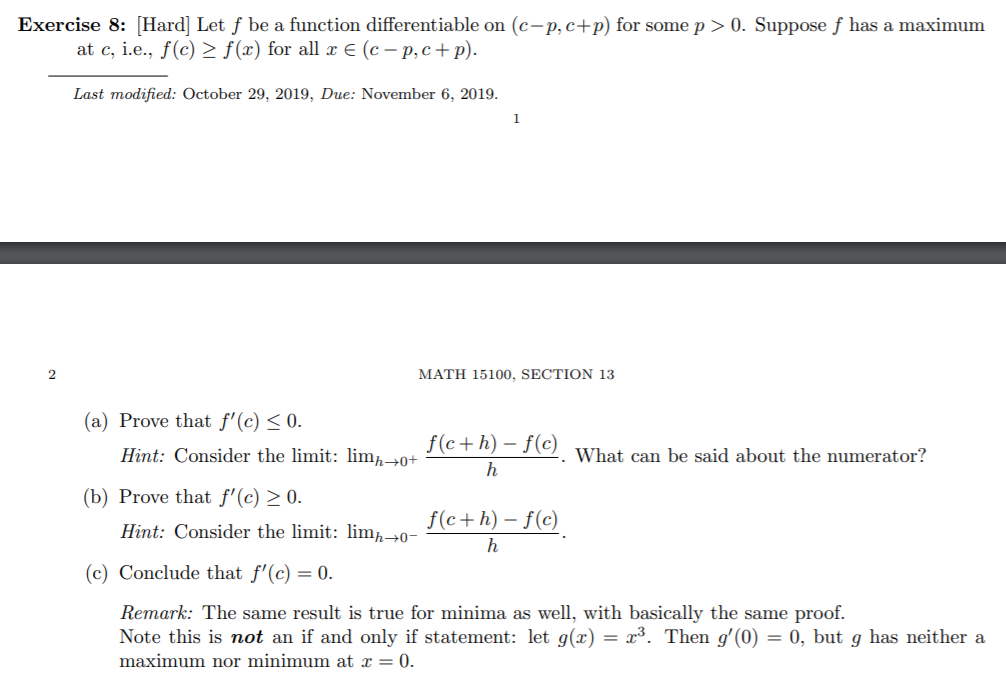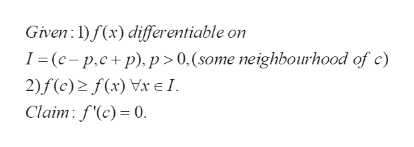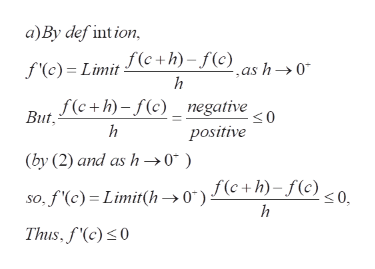# Exercise 8:[Hard] Let f be a function differentiable on (c-p, c+p) for some p > 0. Suppose f has a maximumat c, ie., f(c)2 f(x) for all x € (c - p,c+ p)Last modified: October 29, 2019, Due: November 6, 2019.1ΜΑΤΗ 15100, SECTION 132(a) Prove that f'(c) < 0.f(c+h)-f(c)Hint: Consider the limit: lim0+What can be said about the numerator?(b) Prove that f'(c) > 0.f(c+h)-f(c)Hint: Consider the limit: lim/0-(c) Conclude that f'(c) = 0.Remark: The same result is true for minima as well, with basically the same proof.Note this is not an if and only if statement: let g(x) = x3. Then g'(0) = 0, but g has neither amaximum nor minimum at x = 0

Question
9 viewshelp_outlineImage TranscriptioncloseExercise 8:[Hard] Let f be a function differentiable on (c-p, c+p) for some p > 0. Suppose f has a maximum at c, ie., f(c)2 f(x) for all x € (c - p,c+ p) Last modified: October 29, 2019, Due: November 6, 2019. 1 ΜΑΤΗ 15100, SECTION 13 2 (a) Prove that f'(c) < 0. f(c+h)-f(c) Hint: Consider the limit: lim0+ What can be said about the numerator? (b) Prove that f'(c) > 0. f(c+h)-f(c) Hint: Consider the limit: lim/0- (c) Conclude that f'(c) = 0. Remark: The same result is true for minima as well, with basically the same proof. Note this is not an if and only if statement: let g(x) = x3. Then g'(0) = 0, but g has neither a maximum nor minimum at x = 0 fullscreen
check_circle

Step 1

To prove that the derivative of f(x) vanishes at any critical point x=c (maxmum or minimum)

Step 2

Under the given conditions, the derivative vanishes at a maximum pointhelp_outlineImage TranscriptioncloseGiven )f(x) differentiable on I (c-p.c+ p), p> 0.(some neighbourhood of c) 2)f(c)2 f(x) V I Claim: f'(c) = 0. fullscreen
Step 3

a) the derivativ f'(c) is less than or equal to 0. As the limit of the difference quotient exists (as f is ...help_outlineImage Transcriptionclosea)By def int ion, f'(e) Limitc+h)- f(c) h ,as h->0* f(c+h)-f(c) negative But h positive (by (2) and as h0* so,f'(c) Limit(h->0) (c+h)-f(©) <0, h Thus, f'(c)0 fullscreen

### Want to see the full answer?

See Solution

#### Want to see this answer and more?

Solutions are written by subject experts who are available 24/7. Questions are typically answered within 1 hour.*

See Solution
*Response times may vary by subject and question.
Tagged in

### Math